# VSEPR Theory - Shapes of Molecules

## Molecular Geometry - Linear, Trigonal Planar

Atoms come together to form molecules and molecules come together and bind by chemicals bonds to form elements or compounds. Sometimes atoms of particular elements easily bond with different atoms to form molecules. Chemical bonds can result from three types of interactions between atoms:

1) Electron exchange.
2) Sharing
3) Co-ordinate

The strength of a chemical bond varies from strong or primary bonds and weak or secondary bonds. Strong chemical bonding is due to the sharing or transfer of electrons between the atoms involved. Quantum theory explains all kinds of bonding, but the simpler explanation is given by octet rule and VSEPR theory. Electron sharing involves the “sharing” of one or more electrons between the atoms involved in the bond formation, whereas electron exchange is the exchange of electrons between atoms and not the sharing. Co-ordinate bond is a type of covalent bond where the electrons being shared are contributed from one atom only.

Molecules, as mentioned earlier, are made up of atoms. They have different sizes and structures. They vary in complexity as well. For example, an oxygen molecule is made of two atoms of oxygen, but sometimes under different environmental conditions, oxygen forms a molecule consisting of three atoms of oxygen, called Ozone (O3).

To find the types and number of atomic bonds in a substance and also to show which atoms have lone pairs of electrons, the Lewis electron-pair theory can be used. However, this theory provides no information on how the atoms are arranged. The VSEPR model helps to understand the different shapes and arrangement of molecules. But this model does not say anything regarding the multiple bonds present or the bond length. It is just a representative model.

The VSEPR model is a straightforward yet useful way to understand and explain the shapes and structure of molecules. To reduce the electrostatic repulsion between electron pair is what the theory is based on. Before starting to use the VSEPR model, the Lewis dot picture is considered to determine the electron domain. Electron domain is nothing but the number of bonds and lone pairs in an atom. Knowing this the electron geometry can be obtained. Follow the below steps,

1) Find out the sum of the total number of lone pairs and the number of binding domains.
2) The sum called the steric number determines the electronic shape of the molecule. For example, a steric number of two gives a linear electronic structure.
3) The electronic geometry also determines the angles between the electron domains.
4) The arrangement of angles from highest to lowest is determined by the hierarchy of repulsion where lone pair-lone pair is the highest then comes lone pair-bonding which is slightly lower, and bonding-bonding is the least.

The 3-D shapes and structures of several molecules cannot be determined by Lewis electron-pair theory, and therefore the VSEPR model is used. This model helps in finding the structures of both molecules, having a central metallic atom and a non-metallic central atom. The main proposition of the VSEPR model is that the binding domains and the lone pairs will repel each other and this causes them to take up a geometry that separates each other as far apart as possible.

According to this theory, the valence electrons in an atom can form a single bond, a double bond, a triple bond, a lone pair of electrons, or even a single unpaired electron and is counted as a lone pair. The arrangement of electron groups where the electron repulsion is minimized is the most stable arrangement. A molecule or polyatomic ion is represented as AXmEn. Here A is the central atom, X is the bonded atom, and E is the nonbonding valence electron with m and n being integers. Groups around the central atom are present as bonding pair (BP) or lone pair (LP). The interactions of these two will help predict the positions of the atoms and the bond angles.

How to predict molecular geometry using VSEPR?

VSEPR procedure to predict the molecular geometry:
1) Draw the Lewis electron-pair structure
2) Find the electron group present around the central atom which reduces the repulsion
3) Assign AXmEn
4) Explain the molecular geometry obtained

(This procedure is better understood with the help of examples mentioned below showing different molecular geometry)

1. CO2
A molecule which has two bonded atoms and no lone pair of electrons (around the central atom): This is an example of AX2.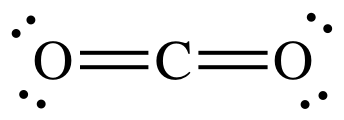In this molecule, Carbon is contributing 4 electrons for bond formation, and each oxygen atom is donating a pair of electrons. Both the electron groups around the central atom, i.e. Carbon are BP. The model focuses only on the central atom and hence the lone pair of electrons on the oxygen atoms does not play a role in determining the molecular geometry.

In this case to reduce repulsion the molecular geometry is linear.

2. BF3
A molecule which has three bonded atoms and no lone pair of electrons: This is an example of AX3.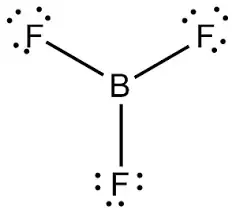Boron donates three electrons, and fluorine has seven valence electrons. Here, the molecular geometry is a trigonal planar.

3. NH3

A molecule which has three bonded atoms and one lone pair of the electron (around the central atom): This is an example of AX3E.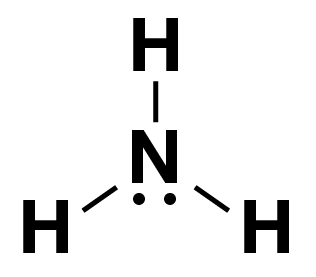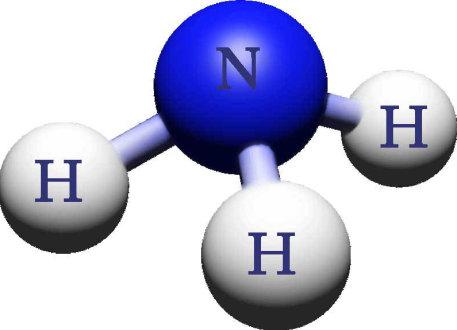In this molecule, NH3 has four electron groups, three BP and one LP.

Nitrogen donates six electrons for bond formation, and each hydrogen atom gives one electron. To reduce repulsion in this case, trigonal pyramidal is the molecular geometry here.

4. OF2

A molecule which has two bonded atoms and two pairs of lone electrons: This is an example of AX 2E2.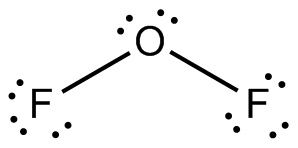In this molecule, oxygen has four electron groups, two BP and two LP.

Oxygen donates two electrons for bond formation, and two pairs are lone pairs. In this case, to reduce the repulsion, the molecular geometry is bent or V-shaped.

5. NH4

A molecule with four bonded atoms and zero pairs of lone electrons: This is an example of AX4.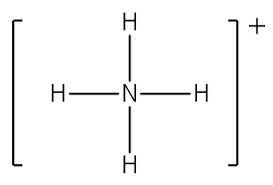In this molecule, ammonia has four valence electrons, and all four are involved in bond formation whereas hydrogen contributes its one electron in bond formation. The molecular geometry observed, in this case, is tetrahedral.

6. PCl5

A molecule with five bonded atoms and zero pairs of lone electrons: This is an example of AX5.

In this molecule, Phosphorus has five electron groups, and all five are BP.
Phosphorus has five electrons which it donates for bond formation, and each chlorine gives a single atom for bond formation. The total valence electrons in phosphorus are five, and in chlorine, there are seven.
The molecular geometry here is trigonal bipyramidal.

7. SF6

A molecule with six bonded atoms and zero pairs of lone electrons: This is an example of AX6.

In this molecule, the sulfur atom contributes six electrons for bonding, and each fluorine atom contributes one electron for bonding. This molecule has six electron groups, and all six are BP. To reduce electronic repulsion, the molecular geometry is octahedral.

8. XeF4

A molecule with four bonded atoms and two pairs of lone electrons: This is an example of AX 4E2.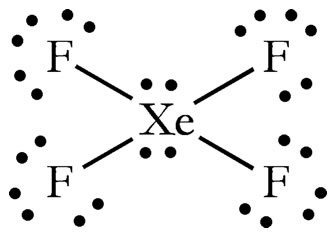In this molecule, Xenon has eight valence electrons, and all four are involved in bond formation whereas the two remaining pair are lone pairs. In this case, it has seven valence electrons. The molecular geometry observed, in this case, is square planar.

The VSEPR model can be used to find out the structures of molecules having no central atoms, which is much more complex in comparison to the structures mentioned above.

IN BRIEF

It can be concluded that the Lewis electron-pair theory cannot be used to find out the structure of molecules and the number of lone pairs in a molecule whereas the VSEPR model is beneficial in determining the structure of molecules. It also tells that the structure that proves to minimize repulsion is the one that has the least energy. , and they can be of two types, bonding pair or lone pair. Based on the interaction between BP and LP one can tell the position of atoms and the bond angle in a molecule and hence determining the molecular geometry.

The dipole moment is the asymmetrical distribution of charge which results in the aligning of molecules in an applied magnetic field and this possible for molecules with polar covalent bonds.